2018-10-29 18:45:30 lgyuWT 阅读数 855

1. 提取感兴趣区，用预先制作的感兴趣区掩模与待处理图想相乘，得到感兴趣图像，感兴趣内图像值保持不变，而区外图像值都为0.
2. 屏蔽作用，用掩模对图像上某些区域作屏蔽，使其不参加处理或不参加处理参数计算，或进队屏蔽区做处理统计。
3. 结构特征提取，用相似性变量或图像匹配方法检测和图像中与淹没相似的结构特征。
4. 特殊形状图像的制作。用选定的图像、图形或物体，对待处理的图像进行遮挡，来控制图像处理的区域或处理过程。用于覆盖特定的特定图像或物体称为掩模或模板。

2014-03-31 11:34:33 Linoi 阅读数 4795

cvtColor的原型如下：

C++: void cvtColor(InputArray src, OutputArray dst, int code, int dstCn=0 )Code：

void MainWindow::on_actionBlackWhite_triggered()
{
cv::Mat bw;
cv::cvtColor(image,bw,CV_BGR2GRAY);
QImage bimg = QImage((const uchar*)(bw.data),bw.cols,bw.rows,QImage::Format_Indexed8);
ui->Imagedisplaylabel->setPixmap(QPixmap::fromImage(bimg));
//ui->Imagedisplaylabel->setScaledContents(true);
//ui->Imagedisplaylabel->resize(ui->Imagedisplaylabel->width(),ui->Imagedisplaylabel->height());

}

Example:References:

Code:

void MainWindow::on_actionSmooth_triggered()
{
cv::Mat Smooth;
cv::namedWindow("Ori");
cv::imshow("Ori",image);
cv::GaussianBlur(image,Smooth,cv::Size(5,5),5);
cv::namedWindow("S");
cv::imshow("S",Smooth);
}

Example:Code:

void MainWindow::on_actionSharpe_triggered()
{
cv::namedWindow("ori_s");
cv::imshow("ori_s",image);
cv::Mat sharpe;
cv::GaussianBlur(image,sharpe,cv::Size(5,5),5);
cv::namedWindow("sharpe");
cv::imshow("sharpe",sharpe);
}

Example:References：

GUIDE TO IMAGE SHARPENING

2017-09-24 16:11:39 u013488089 阅读数 844

2017-10-03 00:33:06 hhy_csdn 阅读数 7839

## 图像处理的MATLAB基础

### 一、MATLAB在图像处理领域的优点

#### 友好的编程语言与操作界面

MATLAB的界面也十分友好，它既支持我们用MATLAB语言编写脚本、函数文件完成特定工作，也支持交互式的操作形式，比如种类丰富的工具箱Toolbox，许多工作动动鼠标即可完成。

### 二、MATLAB图像处理相关函数

#### 读取图片

myimage = imread('newborn.tif');

imread ('D:/dataset/image/newborn.tif');
% 注意语句后面的分号不要省略，否则我们的command window会被庞大无比的像素矩阵充满

#### 显示图片

figure(1);
I = imshow(myimage);
title('newborn.tif');impixelinfo; %注意，pixval on早在MATLAB 2008a就已经淘汰了% 调整背景为绿色
whitebg ('g');
whitebg ('green');
whitebg ([0 1 0]);% 绿色的RGB值
% 其他颜色还有 Cyan blue white magenta yellow red black 等等#### 获取图片信息

size(myimage)% 可以显示矩阵的大小尺寸，几行几列几页

myimage(100,200,2)% 显示第2页第100行第200列的值

impixel(myimage,100,200)% 显示myimage图片在100行200列处的色彩值

imfinfo('name_of_img.jpg');% 可以显示图片的详细信息

impixel的用法

#### 写入图片

% 函数参数格式
imwrite(X,map,'filename','fmt');
% 例子，可以用来转换图片格式，tif->png
imwrite(a,'autumn.png','png');

#### 生成伪彩色图片

% 输入一张灰度图名称，不必键入单引号
im=input('Enter the file name (gray level image) :','s');
% k是读入的照片，灰度图的z=1
[x y z]=size(k);
% 将uint8格式转换为double格式
k=double(k);
% 对灰度图做变换，生成三个色彩通道
for i=1:x
for j=1:y
if k(i,j)>=0 & k(i,j)<50
m(i,j,1)=k(i,j,1)+5;
m(i,j,2)=k(i,j)+10;
m(i,j,3)=k(i,j)+10;
end
if k(i,j)>=50 & k(i,j)<100
m(i,j,1)=k(i,j)+35;
m(i,j,2)=k(i,j)+28;
m(i,j,3)=k(i,j)+10;
end
if k(i,j)>=100 & k(i,j)<150
m(i,j,1)=k(i,j)+52;
m(i,j,2)=k(i,j)+30;
m(i,j,3)=k(i,j)+15;
end
if k(i,j)>=150 & k(i,j)<200
m(i,j,1)=k(i,j)+50;
m(i,j,2)=k(i,j)+40;
m(i,j,3)=k(i,j)+25;
end
if k(i,j)>=200 & k(i,j)<=256
m(i,j,1)=k(i,j)+120;
m(i,j,2)=k(i,j)+60;
m(i,j,3)=k(i,j)+45;
end
end
end
% 显示原图像
figure,imshow(uint8(k),[]);
% 显示生成的伪彩色图片
figure,imshow(uint8(m),[]);


#### 256色、高彩色、真彩色#### MATLAB中常用的数据类型% 示例代码
% 原始图片
figure, imshow(em,emap);
% 索引图转换成灰度图
ygray = ind2gray(em, emap);
figure, imshow (ygray);
% 灰度图转换成索引图
[y, map] = gray2ind(ygray);
figure, imshow (y);
% 读入原始图片
figure,imshow(im);
% rgb图像转灰度图
yim = rgb2gray(im);
figure,imshow(yim);
% 灰度图转RGB图
yim2 = gray2rgb(yim);
figure,imshow(yim2);
% 读入原始图像
% rgb图像转换为128色的索引图
[X,map] = rgb2ind(RGB,128);
figure, imshow(X,map);
% rgb图像转换为256色的索引图
[X,map] = rgb2ind(RGB,256);
figure, imshow(X,map);
% rgb图像转换为2色的索引图
[X,map] = rgb2ind(RGB,2);
figure, imshow(X,map);
% 索引图转换为rgb图
figure, imshow(em,emap)
y = ind2rgb(em,emap)
figure, imshow(y)

### 三、小练习

1）试着在MATLAB的命令窗口键入help imdemos,之后命令窗口会给出Image Processing Toolbox中所有的示例（sample）。列一个表格，看看有多少个样本图片。

Sample TIFF images:
autumn.tif,
board.tif,
cameraman.tif,
canoe.tif,
cell.tif,
circbw.tif,
circuit.tif,
eight.tif,
forest.tif,
kids.tif,
logo.tif,
m83.tif,
moon.tif,
mri.tif,
paper1.tif,
pout.tif,
spine.tif,
tire.tif,
trees.tif.
Binary:
circbw.tif (3822),
logo.tif (1074).

Grayscale:
cameraman.tif (65536),
cell.tif (30369),
circuit.tif (76160),
eight.tif (74536),
moon.tif (192246),
paper1.tif (51296),
pout.tif (69840),
tire.tif (47560).

True color:
autumn.tif (213210),
board.tif (594864),
forest.tif (134547).

Indexed color:
canoe.tif (71622),
forest.tif (134547),
kids.tif (127200),
m83.tif (151200),
mri.tif (16384),
spine.tif (179830),
trees.tif (90300).

2）找一张灰度图，然后利用imwrite()函数将其写为JPEG、PNG、BMP格式的图片，并且比较他们各自的大小。

2019-04-30 10:42:49 qq_33369187 阅读数 647

# 图像处理与识别真彩色增强

## 一、真彩色增强原理

HSI模型反应了人的视觉系统观察彩色的方式，使用非常接近于人对彩色感知的方式来定义彩色。对于图像处理来说，这种模型的优势在于将颜色信息和灰度信息分开了。色调(Hue)分量是描述一种纯色的颜色属性(如红色，绿色，黄色)，饱和度(Saturation)分量是一种纯色被白光稀释的程度的度量，也可以理解为颜色的浓淡程度(如深红色，淡绿色)，亮度(Instensity)分量描述颜色的亮暗程度。这个模型的建立基于以下两个重要事实：
（1） I分量与图像的色彩信息无关
（2） H和S分量与人感受颜色的方式紧密相连

## 二、基础知识

### 1、RGB到HSI转换

RGB 向HSI 模型的转换是由一个基于笛卡尔直角坐标系的单位立方体向基于圆柱极坐标的双锥体的转换。基本要求是将RGB 中的亮度因素分离，将色度分解为色调和饱和度，并用角向量表示色调，如图1所示。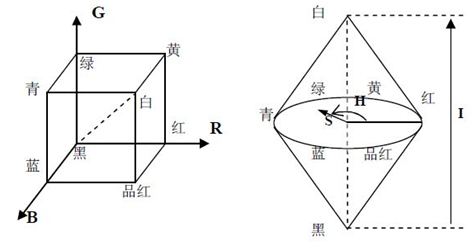RGB转换至HSI：

HSI模型采用三棱锥还是圆锥或者圆柱都没有影响，因为它们之间可以直接相互映射，所以三者是等价的。这里用三角形来证明。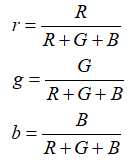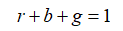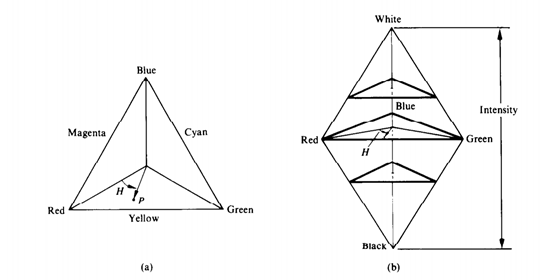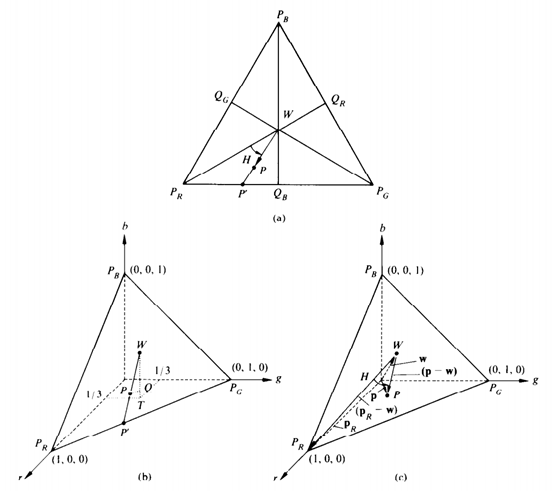### 2、HSI到RGB转换

RG扇形（0<H<120）:当H位于这一扇形区时，RGB分量由下式给出：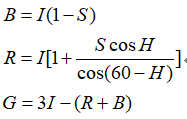GB扇区（120<H<240）：如果给定的H值在这一扇区，首先从H中减去120，即：H=H-120。RGB分量为：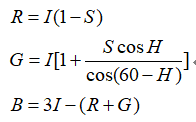BR扇区（240<H<360）:最后，如果H在这一扇区，从H中减去240：H=H-240。RGB分量为：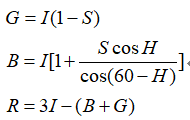### 3、灰度变换增强的方法

（1）线性灰度变换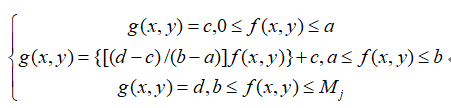（2）分段线性灰度变换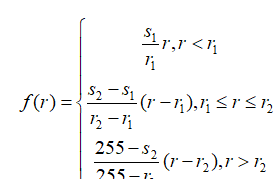（3）对数函数灰度变换

## 三、真彩色增强方法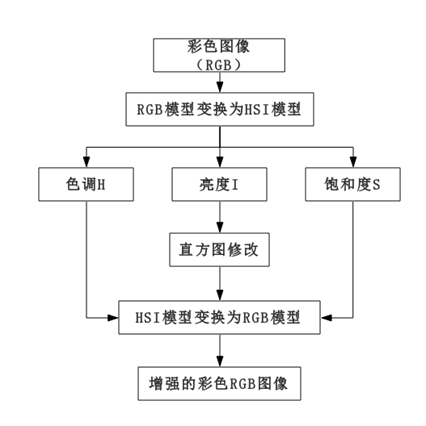### 1、对HSI图像亮度增强

（1）将RGB图转化为HSI图；
（2）利用对灰度图增强的方法增强其中的I分量；
（3）再将结果转化为用RGB图来显示。

### 2、对HSI图像饱和度增强

（1）将RGB图转化为HSI图；
（2）增强其中的S分量；
（3）再将结果转化为用RGB图来显示。

### 3、对HSI图像饱和度与亮度增强

（1）将RGB图转化为HSI图；
（2）增强其中的I与S分量；
（3）再将结果转化为用RGB图来显示。

### 4、直接对RGB图像增强

（1）将RGB图像分解为R、G、B三分量图；
（2）分别对R、G、B三基色进行直方图修改；
（3）增强后再合成RGB图像。

## 四、程序设计

### 1、对HSI图像线性变换增强亮度

var foo = ‘bar’;

clear;clc;
hsi = rgbtohsi(rgb);
H = hsi(:,:,1);
S = hsi(:,:,2);
I = hsi(:,:,3);
I =I*1.4;
hsi_new = cat(3,H,S,I);
rgb_new = hsitorgb(hsi_new);
rgb_new = min(rgb_new,1);
figure;
subplot(221);imshow(rgb)
title('原始rgb图像')
subplot(222);imshow(hsi)
title('hsi图像')
subplot(223);imshow(rgb_new)
title('线性变换增强亮度后的rgb图像')
subplot(224);imshow(hsi_new)
title('增强后的hsi图像')


### 2、对HSI图像分段线性变换增强亮度

var foo = ‘bar’;

clear;clc;
hsi = rgbtohsi(rgb);
H = hsi(:,:,1);
S = hsi(:,:,2);
I = hsi(:,:,3);
if I<50
I=I*1.1;
end
if 50<=I<=200;
I=I*1.4;
end
if I>=200;
I=I*1.1;
end
hsi_new = cat(3,H,S,I);
rgb_new = hsitorgb(hsi_new);
rgb_new = min(rgb_new,1);
figure;
subplot(221);imshow(rgb_new)
title('分段线性变换增强亮度后的rgb图像')


### 3、对HSI图像线性变换增强对比度

var foo = ‘bar’;

clear;clc;
hsi = rgbtohsi(rgb);
H = hsi(:,:,1);
S = hsi(:,:,2);
I = hsi(:,:,3);
S =S*2.0;
hsi_new = cat(3,H,S,I);
rgb_new = hsitorgb(hsi_new);
rgb_new = min(rgb_new,1);
figure;
subplot(221);imshow(rgb)
title('原始rgb图像')
subplot(222);imshow(hsi)
title('hsi图像')
subplot(223);imshow(rgb_new)
title('仅增强饱和度后的rgb图像')
subplot(224);imshow(hsi_new)
title('增强后的hsi图像')



### 4、对HSI图像对数变换增强对比度

var foo = ‘bar’;

clear;clc;
hsi = rgbtohsi(rgb);
H = hsi(:,:,1);
S = hsi(:,:,2);
I = hsi(:,:,3);
if S<50
S=S*1.5;
end
if 50<=S<=200;
S=S*2.0;
end
if S>=200;
S=S*1.5;
end
hsi_new = cat(3,H,S,I);
rgb_new = hsitorgb(hsi_new);
rgb_new = min(rgb_new,1);
figure;
subplot(221);imshow(rgb_new)
title('分段线性变换增强饱和度后的rgb图像')


### 5、对HSI图像线性变换增强饱和度与亮度

var foo = ‘bar’;

clear;clc;
hsi = rgbtohsi(rgb);
H = hsi(:,:,1);
S = hsi(:,:,2);
I = hsi(:,:,3);
S =S*2.0;
I=I*1.4;
hsi_new = cat(3,H,S,I);
rgb_new = hsitorgb(hsi_new);
rgb_new = min(rgb_new,1);
figure;
subplot(221);imshow(rgb)
title('原始rgb图像')
subplot(222);imshow(hsi)
title('hsi图像')
subplot(223);imshow(rgb_new)
title('增强饱和度')
subplot(224);imshow(hsi_new)
title('增强后的hsi图像')


### 6、对HSI图像分段线性变换增强饱和度与亮度

clear;clc;
hsi = rgbtohsi(rgb);
H = hsi(:,:,1);
S = hsi(:,:,2);
I = hsi(:,:,3);
if S<50
S=S*1.5;
end
if 50<=S<=200;
S=S*2.0;
end
if S>=200;
S=S*1.5;
end
if I<50
I=I*1.1;
end
if 50<=I<=200;
I=I*1.4;
end
if I>=200;
I=I*1.1;
end
hsi_new = cat(3,H,S,I);
rgb_new = hsitorgb(hsi_new);
rgb_new = min(rgb_new,1);
figure;
subplot(221);imshow(rgb_new)
title('分段线性变换增强亮度及饱和度后的rgb图像')


### 7、对RGB图像直接线性变换进行彩色增强

clear;clc;
[o p q]=size(rgb);
r(:,:,1)=rgb(:,:,1);
r(:,:,2)=zeros(o,p);
r(:,:,3)=zeros(o,p);
r=r*1.4;
g(:,:,2)=rgb(:,:,2);
g(:,:,1)=zeros(o,p);
g(:,:,3)=zeros(o,p);
g=g*1.4;
b(:,:,3)=rgb(:,:,3);
b(:,:,2)=zeros(o,p);
b(:,:,1)=zeros(o,p);
b=b*1.4;
rgb_new(:,:,1)=r(:,:,1);
rgb_new(:,:,2)=g(:,:,2);
rgb_new(:,:,3)=b(:,:,3);
figure;
subplot(121);imshow(rgb);title('原始rgb图像');
%subplot(231);imshow(r);title('红色分量图像');
%subplot(232);imshow(g);title('绿色分量图像');
%subplot(233);imshow(b);title('蓝色分量图像');
subplot(122);imshow(rgb_new);title('增强后rgb图像');


### 8、对RGB图像直接分段线性变换进行彩色增强

clear;clc;
[o p q]=size(rgb);
r(:,:,1)=rgb(:,:,1);
r(:,:,2)=zeros(o,p);
r(:,:,3)=zeros(o,p);
g(:,:,2)=rgb(:,:,2);
g(:,:,1)=zeros(o,p);
g(:,:,3)=zeros(o,p);
b(:,:,3)=rgb(:,:,3);
b(:,:,2)=zeros(o,p);
b(:,:,1)=zeros(o,p);
rgb_new(:,:,1)=r(:,:,1);
rgb_new(:,:,2)=g(:,:,2);
rgb_new(:,:,3)=b(:,:,3);
figure;
subplot(221);imshow(rgb_new);title('分段线性变换增强后rgb图像');


# 欢迎使用Markdown编辑器

## 新的改变

1. 全新的界面设计 ，将会带来全新的写作体验；
2. 在创作中心设置你喜爱的代码高亮样式，Markdown 将代码片显示选择的高亮样式 进行展示；
3. 增加了 图片拖拽 功能，你可以将本地的图片直接拖拽到编辑区域直接展示；
4. 全新的 KaTeX数学公式 语法；
5. 增加了支持甘特图的mermaid语法1 功能；
6. 增加了 多屏幕编辑 Markdown文章功能；
7. 增加了 焦点写作模式、预览模式、简洁写作模式、左右区域同步滚轮设置 等功能，功能按钮位于编辑区域与预览区域中间；
8. 增加了 检查列表 功能。

H2O is是液体。

210 运算结果是 1024.

## 如何插入一段漂亮的代码片

// An highlighted block
var foo = 'bar';


• 项目
• 项目
• 项目
1. 项目1
2. 项目2
3. 项目3
• 计划任务
• 完成任务

## 创建一个表格

### SmartyPants

SmartyPants将ASCII标点字符转换为“智能”印刷标点HTML实体。例如：

TYPE ASCII HTML
Single backticks 'Isn't this fun?' ‘Isn’t this fun?’
Quotes "Isn't this fun?" “Isn’t this fun?”
Dashes -- is en-dash, --- is em-dash – is en-dash, — is em-dash

## 创建一个自定义列表

Markdown
Text-to-HTML conversion tool
Authors
John
Luke

## 注释也是必不可少的

Markdown将文本转换为 HTML

## KaTeX数学公式

Gamma公式展示 $\Gamma(n) = (n-1)!\quad\forall n\in\mathbb N$ 是通过欧拉积分

$\Gamma(z) = \int_0^\infty t^{z-1}e^{-t}dt\,.$

## 新的甘特图功能，丰富你的文章

• 关于 甘特图 语法，参考 这儿,

## UML 图表

• 关于 Mermaid 语法，参考 这儿,

## FLowchart流程图

• 关于 Flowchart流程图 语法，参考 这儿.

1. 注脚的解释 ↩︎